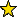# ExteriorModules -- a package for working with modules over exterior algebra

## Description

ExteriorModules is a package for creating and manipulating modules over exterior algebra

Let $K$ be a field, $E$ the exterior algebra of a finite dimensional, $K$-vector space, and $F$ a finitely generated graded free $E$-module with homogeneous basis $g_1, \ldots, g_r$ such that $\mathrm{deg}(g_1) \le \mathrm{deg}(g_2) \le \cdots \le \mathrm{deg}(g_r)$. We present a Macaulay2 package to manage some classes of monomial submodules of $F$. The package is an extension of the one on monomial ideals, and contains some algorithms for computing stable, strongly stable and lexicograhic $E$-submodules of $F$. Such a package also includes some methods to check whether a sequence of nonnegative integers is the Hilbert function of a graded $E$-module of the form $F/M$, with $M$ graded submodule of $F$. Moreover, if $H_{F/M}$ is the Hilbert function of a graded $E$-module $F/M$, some routines are able to compute the unique lexicograhic submodule $L$ of $F$ such that $H_{F/M} = H_{F/L}.$

## CertificationVersion 1.0 of this package was accepted for publication in volume 11 of The Journal of Software for Algebra and Geometry on 3 June 2021, in the article ExteriorModules: a package for computing monomial modules over an exterior algebra. That version can be obtained from the journal or from the Macaulay2 source code repository.

## Version

This documentation describes version 1.0 of ExteriorModules.

## Source code

The source code from which this documentation is derived is in the file ExteriorModules.m2.

## Exports

• Functions and commands
• almostLexModule -- compute an almost lex module with the same Hilbert sequence of the module in input
• almostStableModule -- compute the smallest almost stable module containing a given monomial module
• almostStronglyStableModule -- compute the smallest almost strongly stable module containing a given monomial module
• bassNumbers -- compute the Bass numbers of a given graded module
• createModule -- create a module over an exterior algebra from a list of ideals in input
• getIdeals -- get component ideals from a monomial module
• initialModule -- compute the initial module of a given module
• isAlmostLexModule -- whether a monomial module over an exterior algebra is almost lex
• isAlmostStableModule -- whether a monomial module over an exterior algebra is almost stable
• isAlmostStronglyStableModule -- whether a monomial module over an exterior algebra is almost strongly stable
• isLexModule -- whether a monomial module over an exterior algebra is lex
• isMonomialModule -- whether a module is monomial
• isStableModule -- whether a monomial module over an exterior algebra is stable
• isStronglyStableModule -- whether a monomial module over an exterior algebra is strongly stable
• lexModule -- compute the lex submodule with a given Hilbert sequence in a free module
• lexModuleBySequences -- alternative algorithm to compute the lex submodule with a given Hilbert sequence in a free module
• stableModule -- compute the smallest stable module containing a given monomial module
• stronglyStableModule -- compute the smallest strongly stable module containing a given monomial module
• Methods
• "almostLexModule(Module)" -- see almostLexModule -- compute an almost lex module with the same Hilbert sequence of the module in input
• "almostStableModule(Module)" -- see almostStableModule -- compute the smallest almost stable module containing a given monomial module
• "almostStronglyStableModule(Module)" -- see almostStronglyStableModule -- compute the smallest almost strongly stable module containing a given monomial module
• "bassNumbers(Module)" -- see bassNumbers -- compute the Bass numbers of a given graded module
• "createModule(List,Module)" -- see createModule -- create a module over an exterior algebra from a list of ideals in input
• "getIdeals(Module)" -- see getIdeals -- get component ideals from a monomial module
• hilbertSequence(Module) -- compute the Hilbert sequence of a given module over an exterior algebra
• "initialModule(Module)" -- see initialModule -- compute the initial module of a given module
• "isAlmostLexModule(Module)" -- see isAlmostLexModule -- whether a monomial module over an exterior algebra is almost lex
• "isAlmostStableModule(Module)" -- see isAlmostStableModule -- whether a monomial module over an exterior algebra is almost stable
• "isAlmostStronglyStableModule(Module)" -- see isAlmostStronglyStableModule -- whether a monomial module over an exterior algebra is almost strongly stable
• isHilbertSequence(List,Module) -- whether the given sequence is a Hilbert sequence
• "isLexModule(Module)" -- see isLexModule -- whether a monomial module over an exterior algebra is lex
• "isMonomialModule(Module)" -- see isMonomialModule -- whether a module is monomial
• "isStableModule(Module)" -- see isStableModule -- whether a monomial module over an exterior algebra is stable
• "isStronglyStableModule(Module)" -- see isStronglyStableModule -- whether a monomial module over an exterior algebra is strongly stable
• "lexModule(List,Module)" -- see lexModule -- compute the lex submodule with a given Hilbert sequence in a free module
• "lexModule(Module)" -- see lexModule -- compute the lex submodule with a given Hilbert sequence in a free module
• "lexModuleBySequences(List,Module)" -- see lexModuleBySequences -- alternative algorithm to compute the lex submodule with a given Hilbert sequence in a free module
• minimalBettiNumbers(Module) -- compute the minimal Betti numbers of a given graded module
• "stableModule(Module)" -- see stableModule -- compute the smallest stable module containing a given monomial module
• "stronglyStableModule(Module)" -- see stronglyStableModule -- compute the smallest strongly stable module containing a given monomial module

## For the programmer

The object ExteriorModules is .